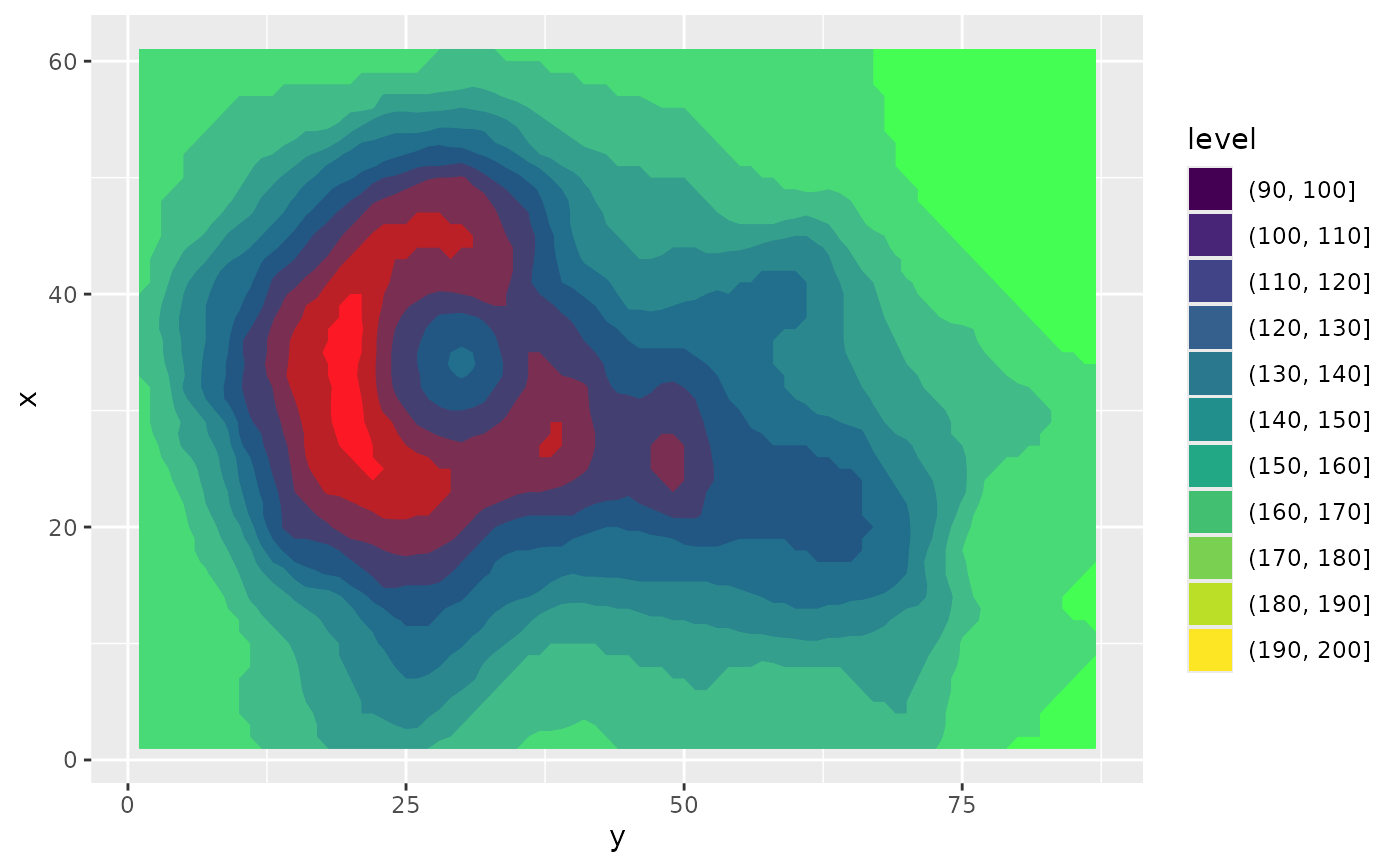Some effects uses a particular channel for specific parameters, such as with_displacement(), which grabs the relative x and y displacements from different channels in some other layer. To facilitate specifying which channel to use from a layer (which is always multichannel), you can wrap the specification in a channel specifier given below. If a filter requires a specific channel and none is specified it will default to luminance (based on the hcl colour space)

ch_red(x, colourspace = "sRGB", invert = FALSE)

ch_green(x, colourspace = "sRGB", invert = FALSE)

ch_blue(x, colourspace = "sRGB", invert = FALSE)

ch_alpha(x, colourspace = "sRGB", invert = FALSE)

ch_hue(x, colourspace = "HCL", invert = FALSE)

ch_chroma(x, colourspace = "HCL", invert = FALSE)

ch_luminance(x, colourspace = "HCL", invert = FALSE)

ch_saturation(x, colourspace = "HSL", invert = FALSE)

ch_lightness(x, colourspace = "HSL", invert = FALSE)

ch_cyan(x, colourspace = "CMYK", invert = FALSE)

ch_magenta(x, colourspace = "CMYK", invert = FALSE)

ch_yellow(x, colourspace = "CMYK", invert = FALSE)

ch_black(x, colourspace = "CMYK", invert = FALSE)

ch_key(x, colourspace = "CMYK", invert = FALSE)

ch_custom(x, channel, colourspace, invert = FALSE)

## Arguments

x

Any object interpretable as a layer

colourspace

The colourspace the channel should be extracted from.

invert

Should the channel values be inverted before use

channel

The name of a channel in the given colourspace

## Value

x with a channel spec attached

## Examples

library(ggplot2)
volcano_long <- data.frame(
x = as.vector(col(volcano)),
y  = as.vector(row(volcano)),
z = as.vector(volcano)
)

# invert the green channel
ggplot(volcano_long, aes(y, x)) +
as_reference(
geom_contour_filled(aes(z = z, fill = after_stat(level))),
id = 'contours'
) +
as_colourspace(
ch_red('contours'),
ch_green('contours', invert = TRUE),
ch_blue('contours')
)